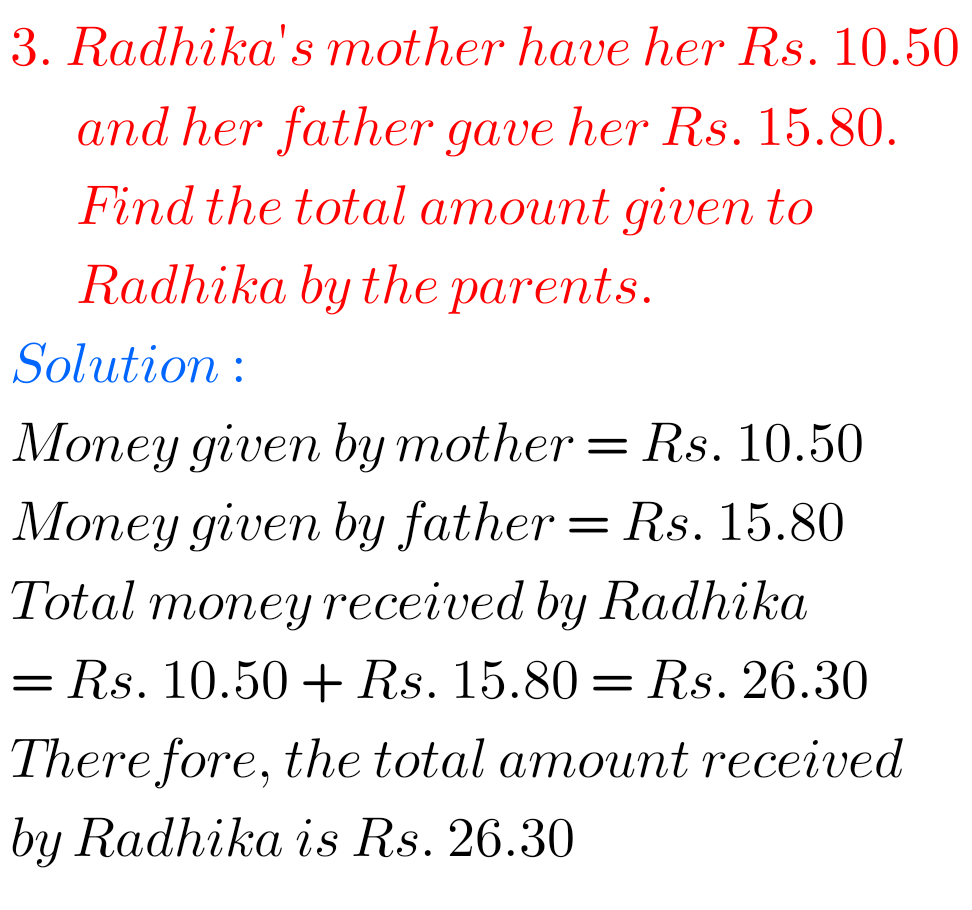## Exercise 8.5 Decimals chapter 8 solutions class 6 Maths Ncert

Decimals chapter 8 exercise 8.5 solutions Ncert maths class 6 Maths Ncert Class 6 textbook Chapter 8 Decimals Exercise 8.5 solutions are given. Study the textbook lesson Decimals very well. Observe the example problems and solutions given in the textbook. Observe the given solutions and try them in your own method. You can see Ncert …